## Wednesday, January 16, 2013

### Length of a Polynomial Segment - Part 1; and the HP 50g Program MPFIT

The next two blog entries are going to deal with finding the length of a polynomial segment.

Polynomial Segments

What is a polynomial segment? I could not find a formal definition, so I'll describe it is this: similar to a line segment where a pair of points are connected with a line, a polynomial segment is a set of points that are connected by polynomial curve. The degree of the polynomial is determined by the number of points, less one. For instance, if we have 3 points, the polynomial that connects them the order 2 (quadratic). A cubic polynomial (order 3) is used to connect a set of 4 points.

The Goal

We have a set of points {(x0, y0), (x1, y1), (x2, y2),... (xn, yn)}. Connect the points using a polynomial. What is the length of that segment?

General Method

1. Find the polynomial to connect the set of points. Order of the elements is important. This is determined by using the matrix calculation (X^T X)^-1 (X^T y) to determine the coefficients. The resulting matrix contains the coefficients of the polynomial; with each term being a coefficient of x of increasing power, from 0 to n-1.

2. Use the arc length formula ∫ ( √ (1 + (f'(x))^2 ) dx, a, b) where a is the minimum of the x values and b is the maximum of the x values.

We recommend using a calculator or math software that can handle matrices and integrals for this procedure.

But what is X and y?

y represents a vector of y-values of the set of points.

X is a Vandermonde Matrix. A Vandermonde matrix is a n × n matrix is formed with each row containing a geometric progression of each term. The first column, each term is raised to the 0th power, the second column each term to the 1st, the third column each term is raised to the second power, and so on until the nth row where each term is raised to the n-1 power.

For instance, a Vandermonde matrix consisting of the set [a, b, c, d] would look like this: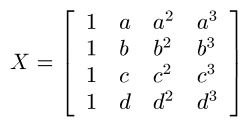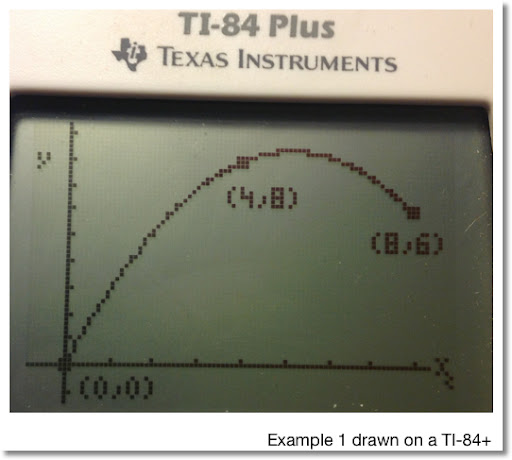Example 1

Find a polynomial segment that fits the points (0,0), (4,8), and (8,6). Find the length of that segment. (See the diagram above).

The x values are [0, 4, 8] with xmin = 0 and xmax = 8. The y values are [0, 8, 6]. Let X be the Vandermonde matrix with the set [0, 4, 8] and the vector y be represented by the corresponding y-values.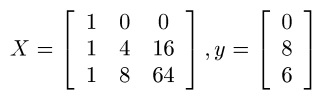Now we need to calculate (X^T X)^-1 (X^T y).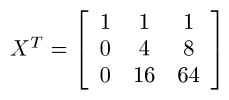Then: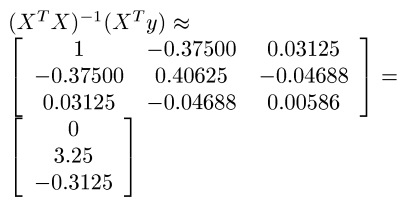The resulting matrix contains the coefficients of the required polynomial. Since the vector has 3 entries, the order of the polynomial is 3-1=2.

Going top-down:
0 is the constant (coefficient of x^0),
3.25 is the coefficient of x,
and -0.3125 is the coefficient of x^2.

Our polynomial is f(x) = 3.25x - 0.03125x^2.

To find the length, use the arc length formula using end points xmin = 0 and xmax = 8. We find that the length of the polynomial segment connecting (0,0), (4,8), and (8,6) is about 14.23920.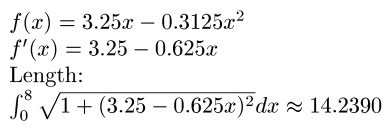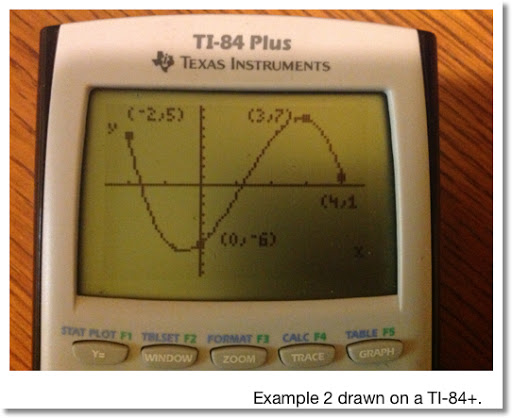Example 2

What is the length of the polynomial segment connected with the points (-2,5), (0,-6), (3,2), and (4,1). We are working with 4 points, the required polynomial has the order 4-1=3. (Cubic polynomial)

The Vandermonde matrix X with the vector y are as follows: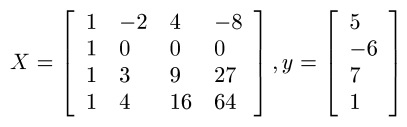Calculating (X^T X)^-1 (X^T y) yields (approximately):

[ [ -6 ]
[ 2.98333 ]
[ 2.725 ]
[ -0.75833 ] ]

To form the cubic polynomial:
-6 is the constant,
2.98333 is the coefficient of x,
2.725 is the coefficient of x^2, and
-0.75833 is the coefficient of x^3.

With xmin = -2 and xmax = 4, we find the length of this segment is approximately 32.60952. (See below).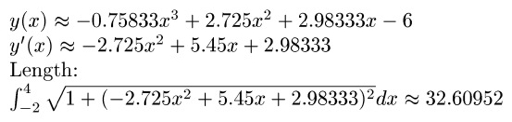HP50g Program MPFIT

The HP 50g program MPFIT fits a polynomial to the set of points {(x0, y0), (x1, y1), (x2, y2), ... , (x_(n-1), y_(n-1))}.

Input:
2: vector of x coordinates
1: vector of y coordinates

Output:
2: Coefficients in matrix form [ [a0] [a1] [a2] ... [a_(n-1)] ]
1: Polynomial of X in the form a0 + a1 * X + a2 * X^2 + ... + a_(n-1) * X^(n-1)

Program MPFIT (size 183.5 bytes)

<< DUP SIZE OBJ→ DROP → MX MY N
<< 'X' PURGE
MY OBJ→ 1 + →ARRY
MX VANDERMONDE
LSQ → MR
<< MR DUP
{1, 1} GET
2 N FOR K
MR K 1 2 →LIST GET
'X' K 1 - ^ * +
NEXT >> >> >>

Example:
Input:
2: [1, 3, 6]
1: [6, 3, 5]

Output:
2: [[8.8] [-3.23333333333] [0.43333333333]]
1: 8.8+-3.23333333333*X+0.43333333333*X^2

Which means the polynomial fit for the given points is (approximately):

8.8 - 3.23333x + 0.43333x^2

(Screen shots of this example are shown below)

To get the length of the segment with the HP 50g, first note the xmin and xmax values, which are 1 and 6, respectively. This keystroke (in RPN Mode) should do the trick:

[ ' ] [ X ] [ENTER]
[RS] [COS] ( δ )
[LS] [ √ ] (x²)
1 [ + ] [ √ ]
1 [ENTER]
6 [ENTER]
3 [LS] [EVAL] (PRG) [F1] (STACK) [NXT] [F1] (ROLL)
[ ' ] [ X ] [ENTER]
[RS] [TAN] ( ∫ )

Length = 7.75662832829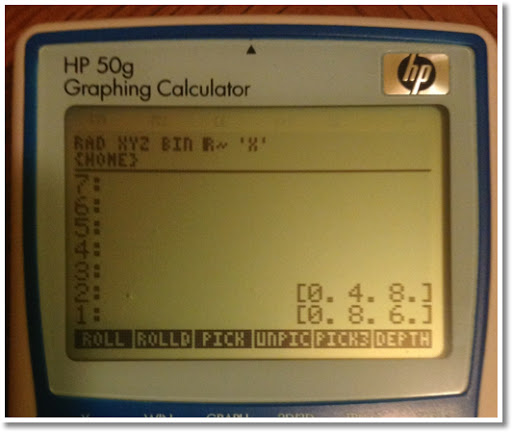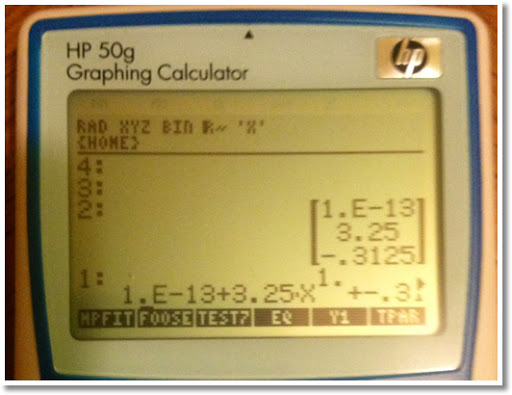My next blog entry will contain programs for the TI-84+ and Casio Prizm (fx series) calculators.

Have a great day everyone,

Eddie

This blog is property of Edward Shore. 2013